Anúncio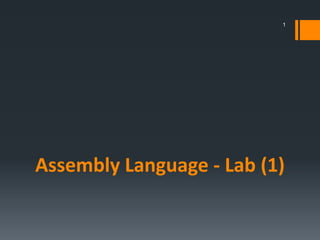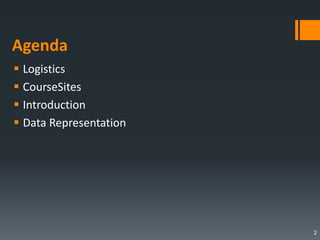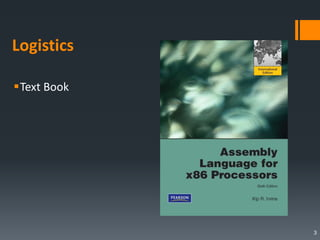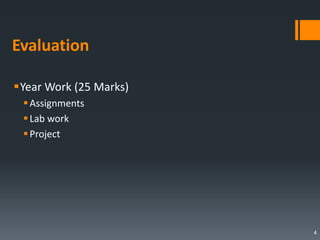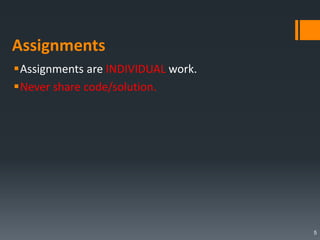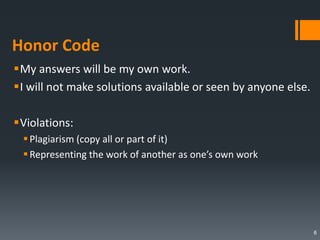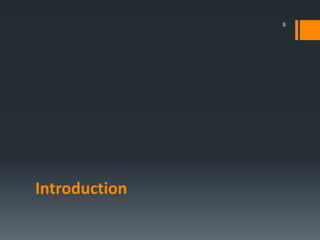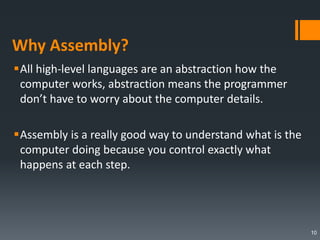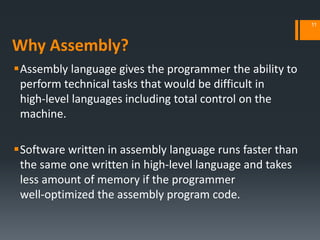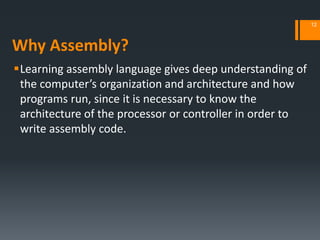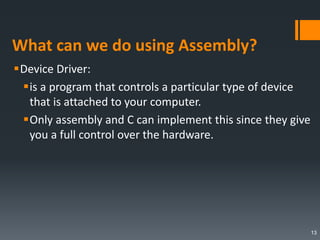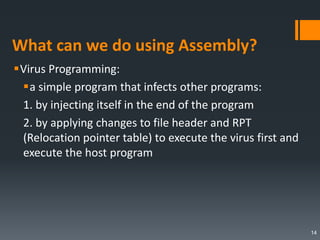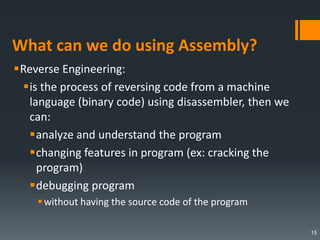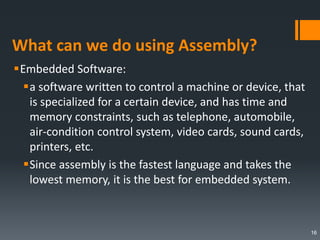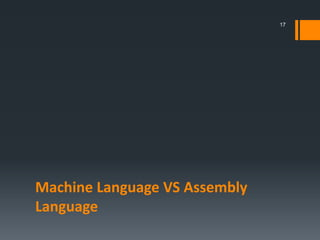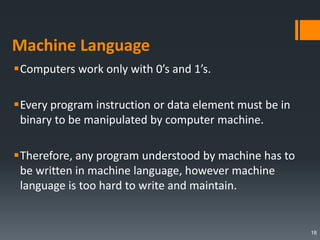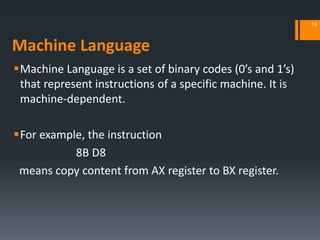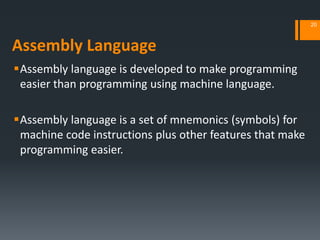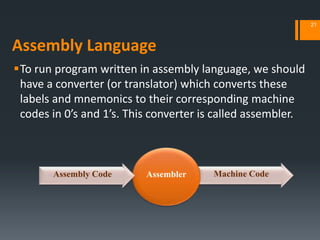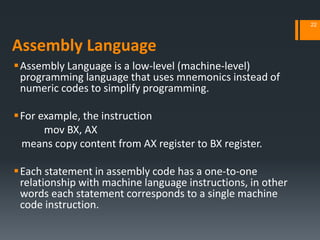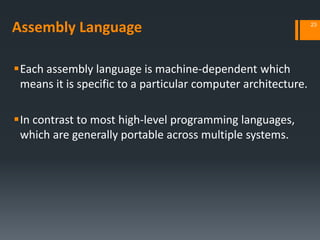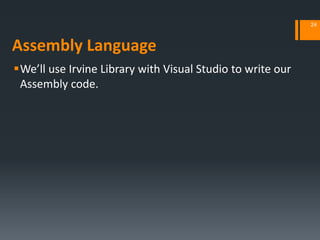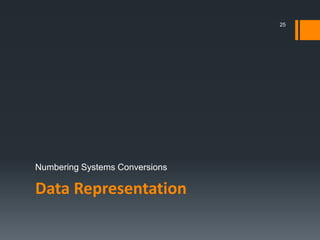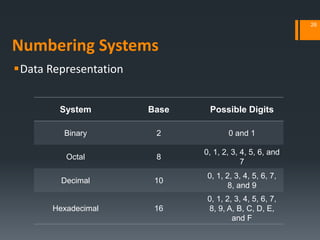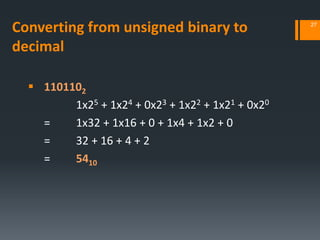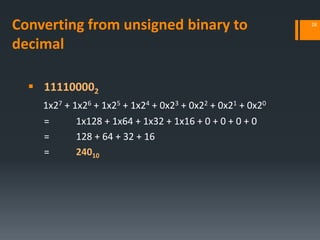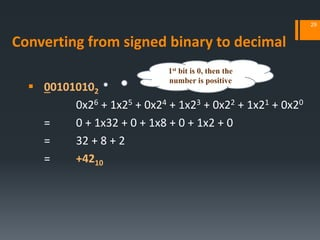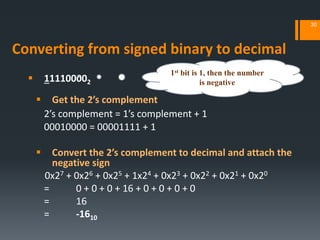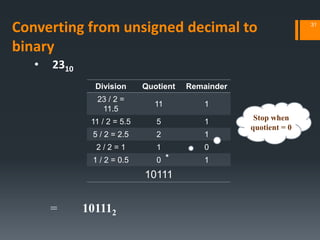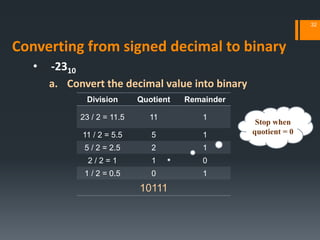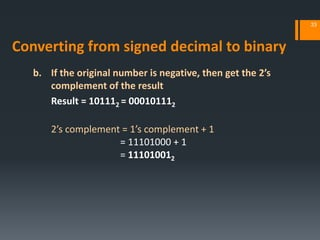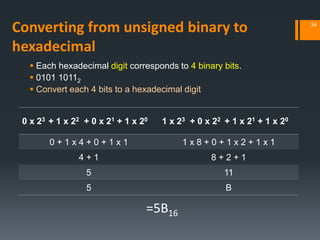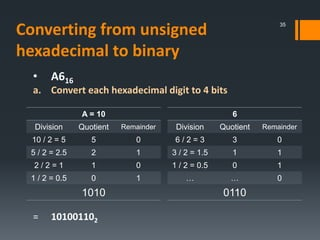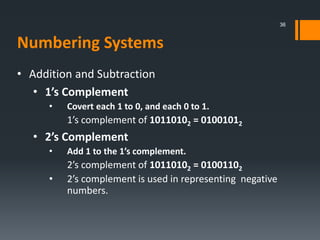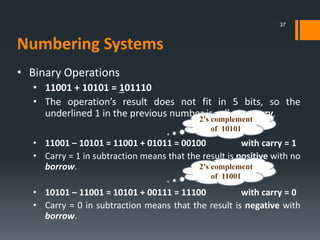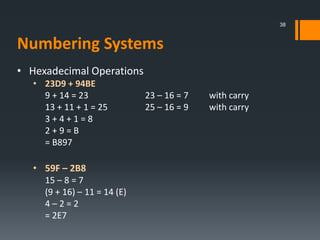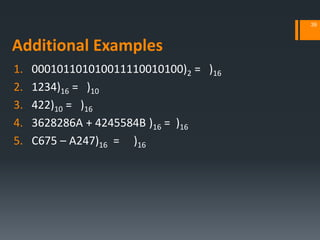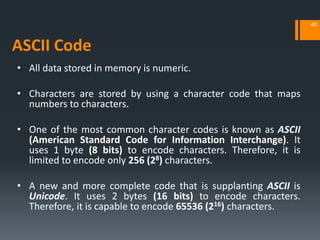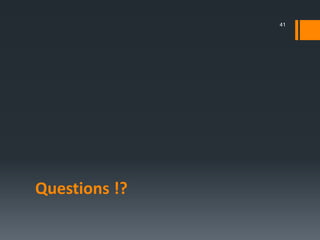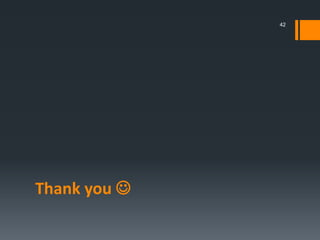1 de 41
Anúncio

### [ASM] Lab1

1. Assembly Language - Lab (1) 1
2. Agenda  Logistics  CourseSites  Introduction  Data Representation 2
3. Logistics Text Book 3
4. Evaluation Year Work (25 Marks) Assignments Lab work Project 4
5. Assignments Assignments are INDIVIDUAL work. Never share code/solution. 5
6. Honor Code My answers will be my own work. I will not make solutions available or seen by anyone else. Violations: Plagiarism (copy all or part of it) Representing the work of another as one’s own work 6
7. CourseSites https://www.coursesites.com/
8. Introduction 9
9. Why Assembly? All high-level languages are an abstraction how the computer works, abstraction means the programmer don’t have to worry about the computer details. Assembly is a really good way to understand what is the computer doing because you control exactly what happens at each step. 10
10. Why Assembly? Assembly language gives the programmer the ability to perform technical tasks that would be difficult in high‐level languages including total control on the machine. Software written in assembly language runs faster than the same one written in high‐level language and takes less amount of memory if the programmer well‐optimized the assembly program code. 11
11. Why Assembly? Learning assembly language gives deep understanding of the computer’s organization and architecture and how programs run, since it is necessary to know the architecture of the processor or controller in order to write assembly code. 12
12. What can we do using Assembly? Device Driver: is a program that controls a particular type of device that is attached to your computer. Only assembly and C can implement this since they give you a full control over the hardware. 13
13. What can we do using Assembly? Virus Programming: a simple program that infects other programs: 1. by injecting itself in the end of the program 2. by applying changes to file header and RPT (Relocation pointer table) to execute the virus first and execute the host program 14
14. What can we do using Assembly? Reverse Engineering: is the process of reversing code from a machine language (binary code) using disassembler, then we can: analyze and understand the program changing features in program (ex: cracking the program) debugging program without having the source code of the program 15
15. What can we do using Assembly? Embedded Software: a software written to control a machine or device, that is specialized for a certain device, and has time and memory constraints, such as telephone, automobile, air-condition control system, video cards, sound cards, printers, etc. Since assembly is the fastest language and takes the lowest memory, it is the best for embedded system. 16
16. Machine Language VS Assembly Language 17
17. Machine Language Computers work only with 0’s and 1’s. Every program instruction or data element must be in binary to be manipulated by computer machine. Therefore, any program understood by machine has to be written in machine language, however machine language is too hard to write and maintain. 18
18. Machine Language Machine Language is a set of binary codes (0’s and 1’s) that represent instructions of a specific machine. It is machine‐dependent. For example, the instruction 8B D8 means copy content from AX register to BX register. 19
19. Assembly Language Assembly language is developed to make programming easier than programming using machine language. Assembly language is a set of mnemonics (symbols) for machine code instructions plus other features that make programming easier. 20
20. Assembly Language To run program written in assembly language, we should have a converter (or translator) which converts these labels and mnemonics to their corresponding machine codes in 0’s and 1’s. This converter is called assembler. 21 Machine CodeAssemblerAssembly Code
21. Assembly Language Assembly Language is a low-level (machine‐level) programming language that uses mnemonics instead of numeric codes to simplify programming. For example, the instruction mov BX, AX means copy content from AX register to BX register. Each statement in assembly code has a one-to-one relationship with machine language instructions, in other words each statement corresponds to a single machine code instruction. 22
22. Assembly Language Each assembly language is machine‐dependent which means it is specific to a particular computer architecture. In contrast to most high-level programming languages, which are generally portable across multiple systems. 23
23. Assembly Language We’ll use Irvine Library with Visual Studio to write our Assembly code. 24
24. Data Representation Numbering Systems Conversions 25
25. Numbering Systems Data Representation 26 System Base Possible Digits Binary 2 0 and 1 Octal 8 0, 1, 2, 3, 4, 5, 6, and 7 Decimal 10 0, 1, 2, 3, 4, 5, 6, 7, 8, and 9 Hexadecimal 16 0, 1, 2, 3, 4, 5, 6, 7, 8, 9, A, B, C, D, E, and F
26. Converting from unsigned binary to decimal  1101102 1x25 + 1x24 + 0x23 + 1x22 + 1x21 + 0x20 = 1x32 + 1x16 + 0 + 1x4 + 1x2 + 0 = 32 + 16 + 4 + 2 = 5410 27
27. Converting from unsigned binary to decimal  111100002 1x27 + 1x26 + 1x25 + 1x24 + 0x23 + 0x22 + 0x21 + 0x20 = 1x128 + 1x64 + 1x32 + 1x16 + 0 + 0 + 0 + 0 = 128 + 64 + 32 + 16 = 24010 28
28. Converting from signed binary to decimal  001010102 0x26 + 1x25 + 0x24 + 1x23 + 0x22 + 1x21 + 0x20 = 0 + 1x32 + 0 + 1x8 + 0 + 1x2 + 0 = 32 + 8 + 2 = +4210 29 1st bit is 0, then the number is positive
29. Converting from signed binary to decimal  111100002  Get the 2’s complement 2’s complement = 1’s complement + 1 00010000 = 00001111 + 1  Convert the 2’s complement to decimal and attach the negative sign 0x27 + 0x26 + 0x25 + 1x24 + 0x23 + 0x22 + 0x21 + 0x20 = 0 + 0 + 0 + 16 + 0 + 0 + 0 + 0 = 16 = -1610 30 1st bit is 1, then the number is negative
30. • 2310 = 101112 Division Quotient Remainder 23 / 2 = 11.5 11 1 11 / 2 = 5.5 5 1 5 / 2 = 2.5 2 1 2 / 2 = 1 1 0 1 / 2 = 0.5 0 1 10111 Stop when quotient = 0 31 Converting from unsigned decimal to binary
31. • -2310 a. Convert the decimal value into binary Division Quotient Remainder 23 / 2 = 11.5 11 1 11 / 2 = 5.5 5 1 5 / 2 = 2.5 2 1 2 / 2 = 1 1 0 1 / 2 = 0.5 0 1 10111 Stop when quotient = 0 32 Converting from signed decimal to binary
32. b. If the original number is negative, then get the 2’s complement of the result Result = 101112 = 000101112 2’s complement = 1’s complement + 1 = 11101000 + 1 = 111010012 33 Converting from signed decimal to binary
33. Converting from unsigned binary to hexadecimal  Each hexadecimal digit corresponds to 4 binary bits.  0101 10112  Convert each 4 bits to a hexadecimal digit =5B16 34 0 x 23 + 1 x 22 + 0 x 21 + 1 x 20 1 x 23 + 0 x 22 + 1 x 21 + 1 x 20 0 + 1 x 4 + 0 + 1 x 1 1 x 8 + 0 + 1 x 2 + 1 x 1 4 + 1 8 + 2 + 1 5 11 5 B
34. • A616 a. Convert each hexadecimal digit to 4 bits = 101001102 A = 10 Division Quotient Remainder 10 / 2 = 5 5 0 5 / 2 = 2.5 2 1 2 / 2 = 1 1 0 1 / 2 = 0.5 0 1 1010 6 Division Quotient Remainder 6 / 2 = 3 3 0 3 / 2 = 1.5 1 1 1 / 2 = 0.5 0 1 … … 0 0110 35 Converting from unsigned hexadecimal to binary
35. • Addition and Subtraction • 1’s Complement • Covert each 1 to 0, and each 0 to 1. 1’s complement of 10110102 = 01001012 • 2’s Complement • Add 1 to the 1’s complement. 2’s complement of 10110102 = 01001102 • 2’s complement is used in representing negative numbers. 36 Numbering Systems
36. • Binary Operations • 11001 + 10101 = 101110 • The operation’s result does not fit in 5 bits, so the underlined 1 in the previous number is called a carry. • 11001 – 10101 = 11001 + 01011 = 00100 with carry = 1 • Carry = 1 in subtraction means that the result is positive with no borrow. • 10101 – 11001 = 10101 + 00111 = 11100 with carry = 0 • Carry = 0 in subtraction means that the result is negative with borrow. 37 2’s complement of 10101 2’s complement of 11001 Numbering Systems
37. • Hexadecimal Operations • 23D9 + 94BE 9 + 14 = 23 23 – 16 = 7 with carry 13 + 11 + 1 = 25 25 – 16 = 9 with carry 3 + 4 + 1 = 8 2 + 9 = B = B897 • 59F – 2B8 15 – 8 = 7 (9 + 16) – 11 = 14 (E) 4 – 2 = 2 = 2E7 38 Numbering Systems
38. Additional Examples 1. 000101101010011110010100)2 = )16 2. 1234)16 = )10 3. 422)10 = )16 4. 3628286A + 4245584B )16 = )16 5. C675 – A247)16 = )16 39
39. • All data stored in memory is numeric. • Characters are stored by using a character code that maps numbers to characters. • One of the most common character codes is known as ASCII (American Standard Code for Information Interchange). It uses 1 byte (8 bits) to encode characters. Therefore, it is limited to encode only 256 (28) characters. • A new and more complete code that is supplanting ASCII is Unicode. It uses 2 bytes (16 bits) to encode characters. Therefore, it is capable to encode 65536 (216) characters. ASCII Code 40
40. Questions !? 41
41. Thank you  42